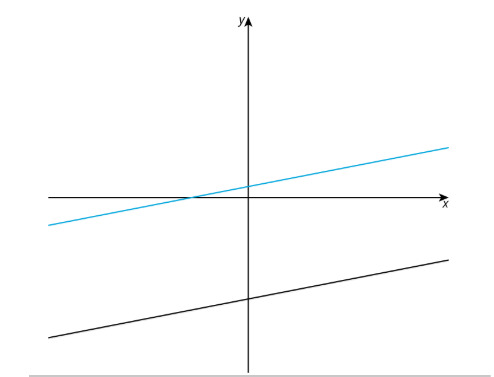PSABPW65 - Cool-Down---Acc7.5 Lesson 14 Solving Systems of Equations (8.EE.C.8)
Part A)Given the lines shown here, what are two possible equations for this system of equations?

Part B)

How many solutions does this system of equations have?